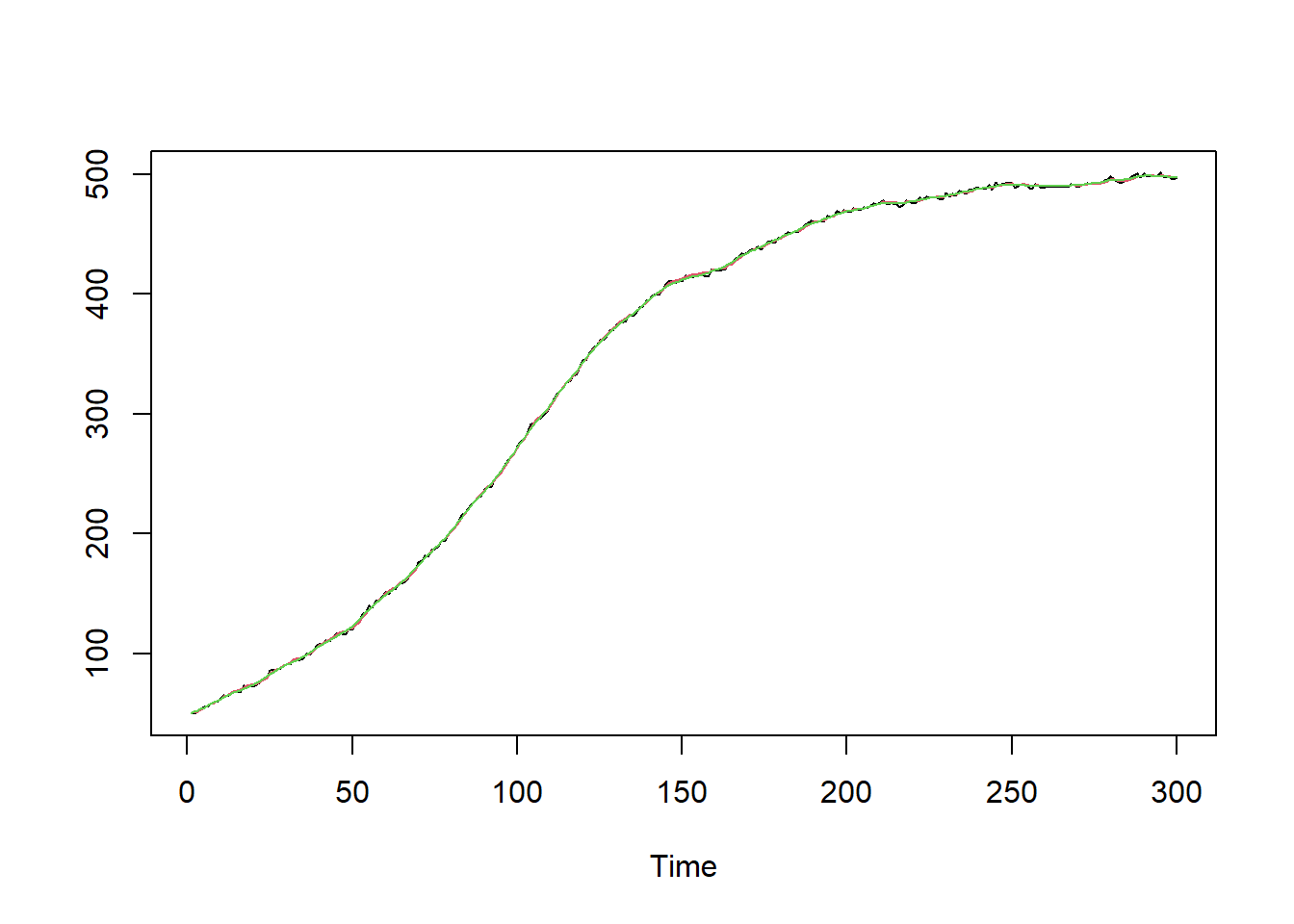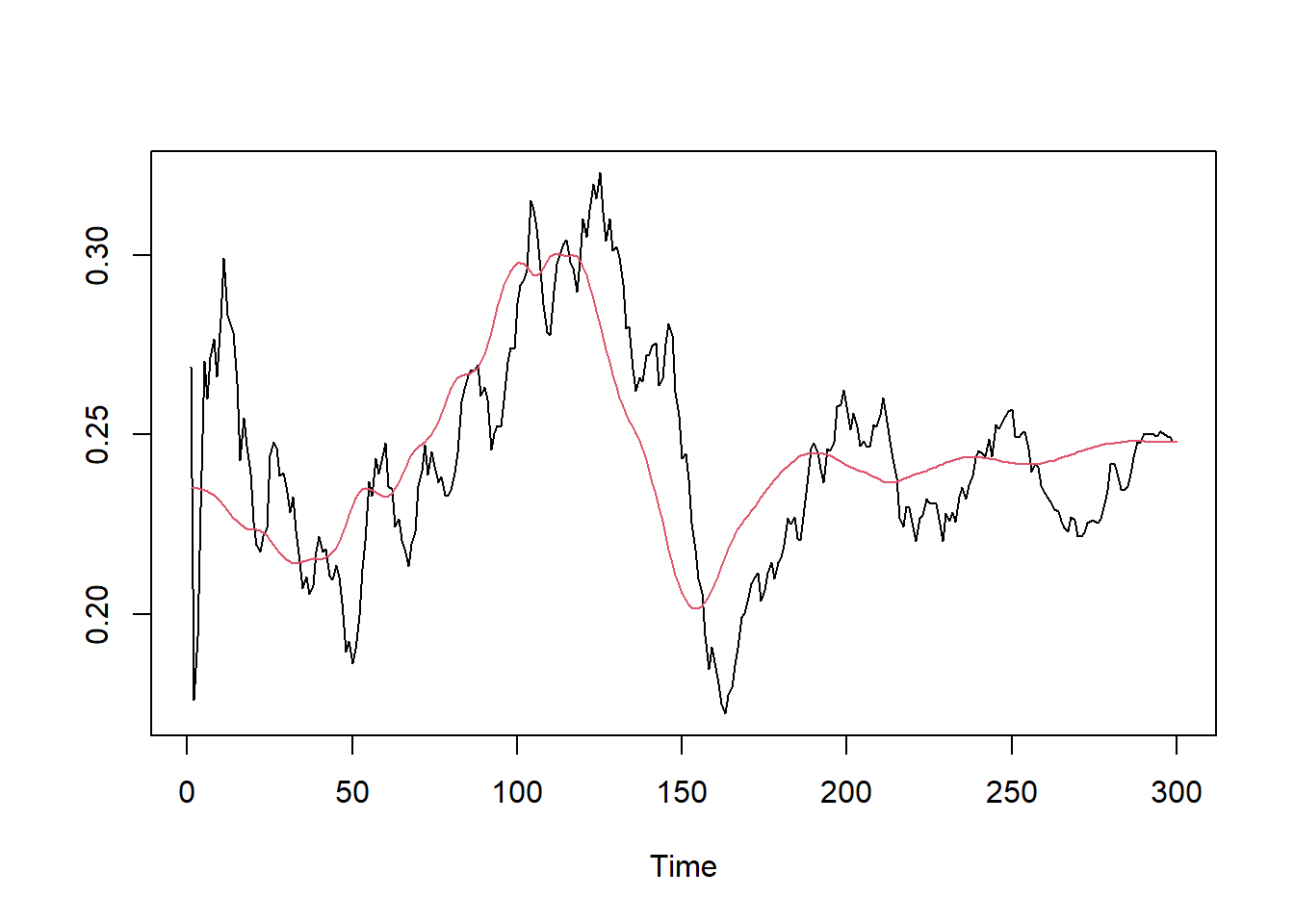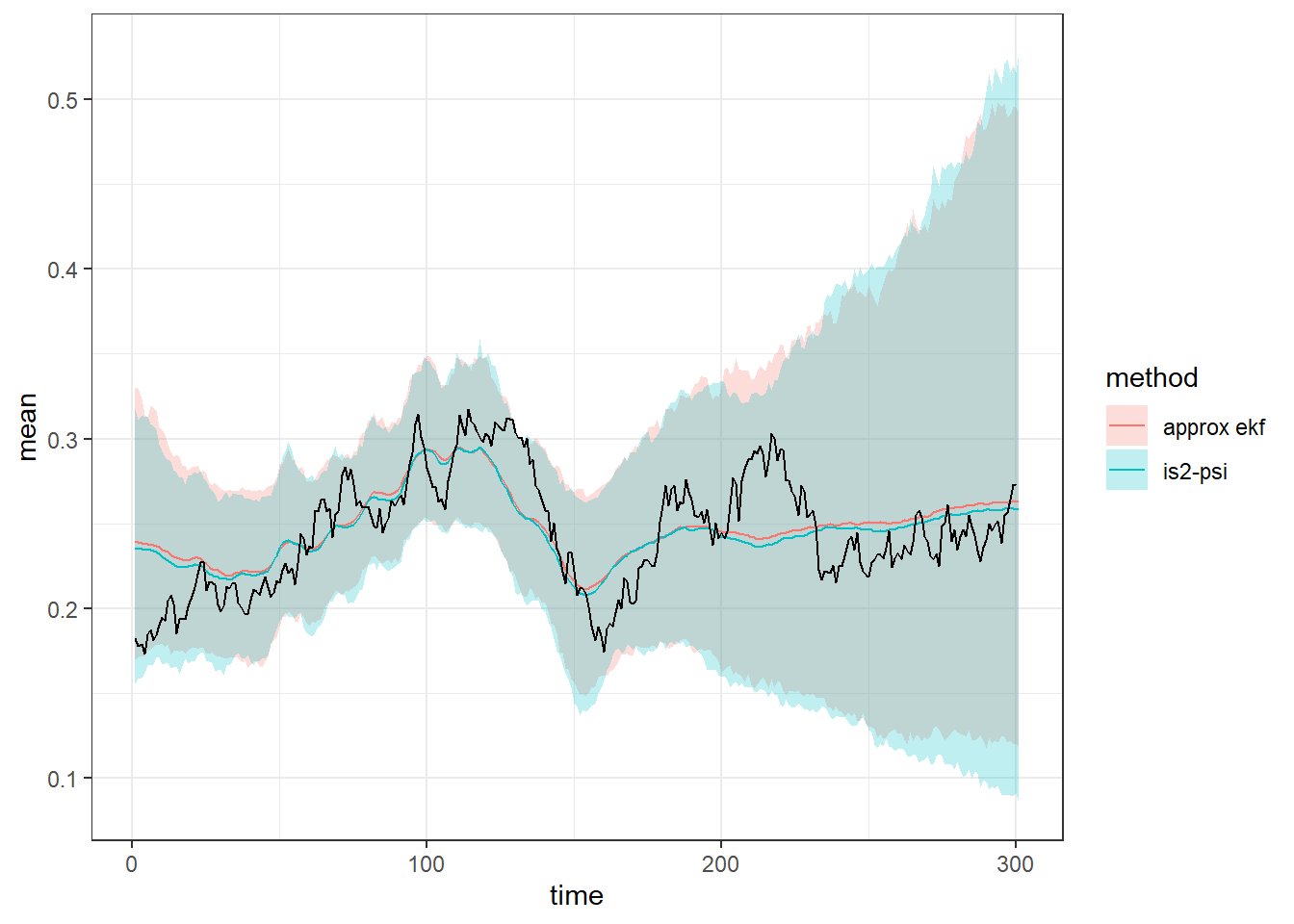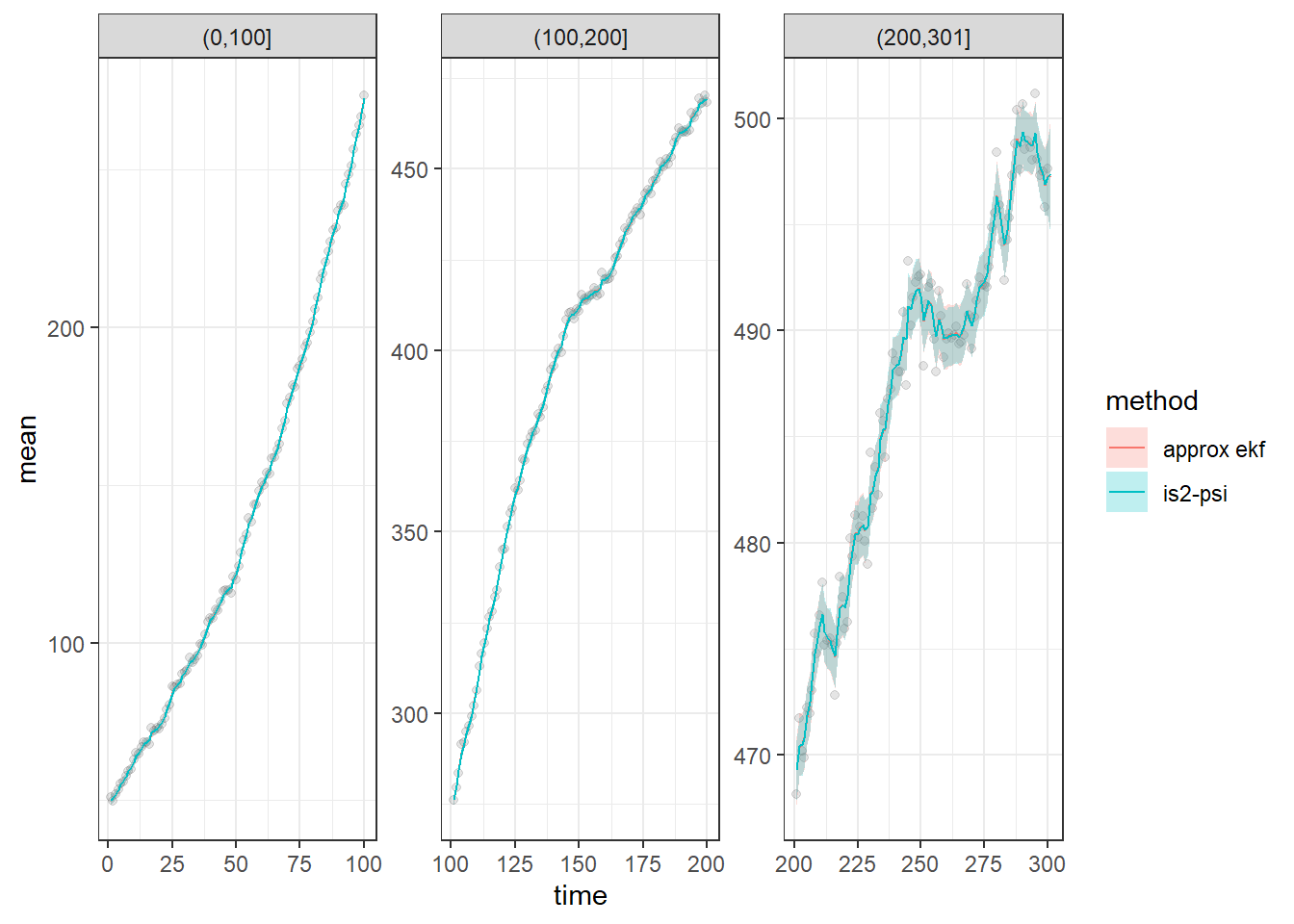## Introduction

This vignette shows how to model general non-linear state space models with bssm. The general non-linear Gaussian model in bssm has following form:

$y_t = Z(t, \alpha_t, \theta) + H(t, \alpha_t, \theta)\epsilon_t,\\ \alpha_{t+1} = T(t, \alpha_t, \theta) + R(t, \alpha_t, \theta)\eta_t,\\ \alpha_1 \sim N(a_1(\theta), P_1(\theta)),$ with $$t=1,\ldots, n$$, $$\epsilon_t \sim N(0,\textrm{I}_p)$$, and $$\eta \sim N(0,\textrm{I}_k)$$. Here vector $$\theta$$ contains the unknown model parameters.

As some of the model matrices may depend on the current state $$\alpha_t$$, constructing for example $$T(t,\alpha_t,\theta)$$ by calling user-defined R function is not feasible, as this should be done repeatedly within the particle filter which would negate the benefits of the whole C++ implementation of the particle filter. Therefore the functions $$T(\cdot)$$, $$H(\cdot)$$, $$T(\cdot)$$, $$R(\cdot)$$,$$a_1(\cdot)$$, $$P_1(\cdot)$$, as well as functions defining the Jacobians of $$Z(\cdot)$$ and $$T(\cdot)$$ and the prior distribution for $$\theta$$ must be defined by user as a external pointers to C++ functions.

As an example, a logistic growth model of form $y_t = p_t + \epsilon_t,\\ p_{t+1} = K p_t \frac{\exp(r_t dt)}{K + p_t (\exp(r_tdt ) - 1)} + \xi_t,\\ r_t = \frac{\exp{r'_t}}{1 + \exp{r'_t}},\\ r'_{t+1} = r'_t + \eta_t,$ with constant carrying capacity $$K = 500$$, initial population size $$p_1 = 50$$, initial growth rate on logit scale $$r'_1 = -1.5$$, $$dt = 0.1$$, $$\xi \sim N(0,1)$$, $$\eta \sim N(0,0.05)$$, and $$\epsilon \sim N(0, 1)$$.

Let’s first simulate some data, with $$\sigma_r=\sigma_p=0$$:

set.seed(1)

p1 <- 50 # population size at t = 1
K <- 500 # carrying capacity
H <- 1 # standard deviation of obs noise

#sample time
dT <- .1

#observation times
t <- seq(0.1, 30, dT)
n <- length(t)
r <- plogis(cumsum(c(-1.5, rnorm(n - 1, sd = 0.05))))
p <- numeric(n)
p <- p1
for(i in 2:n)
p[i] <- rnorm(1, K * p[i-1] * exp(r[i-1] * dT) / (K + p[i-1] * (exp(r[i-1] * dT) - 1)), 1)
# observations
y <- p + rnorm(n, 0, H)

## Model in bssm

The functions determining the model functions are given in file model_functions.cpp. For example, function T_fn defines the state transition function $$T(\cdot)$$:

// [[Rcpp::export]]
arma::vec T_fn(const unsigned int t, const arma::vec& alpha, const arma::vec& theta,
const arma::vec& known_params, const arma::mat& known_tv_params) {

double dT = known_params(0);
double K = known_params(1);

arma::vec alpha_new(2);
alpha_new(0) = alpha(0);
double r = exp(alpha(0)) / (1.0 + exp(alpha(0)));
alpha_new(1) = K * alpha(1) * exp(r * dT) /
(K + alpha(1) * (exp(r * dT) - 1));
return alpha_new;
}

The name of this function does not matter, but it should always return Armadillo vector (arma::vec), and have the same signature (i.e. the order and types of the function’s parameters) should always be like above, even though some of the parameters were not used in the body of the function. Note that all of these functions can also depend on some known parameters, given as known_params (vector) and known_tv_params (matrix) arguments to ssm_nlg function (which are then passed to individual C++ snippets). For details of using Armadillo, see Armadillo documentation. After defining the appropriate model functions, the cpp file should also contain a function for creating external pointers for the aforementioned functions. Why this is needed is more technical issue, but fortunately you can just copy the function from the example file without any modifications.

After creating the file for C++ functions, you need to compile the file using Rcpp1:

Rcpp::sourceCpp("ssm_nlg_template.cpp")
## Warning in normalizePath(path.expand(path), winslash, mustWork): path="C:/
## Users/jovetale/AppData/Local/Temp/RtmpK6bHPg/Rbuild35bc6053b0e/bssm/
## vignettes/../inst/include": The system cannot find the file specified
pntrs <- create_xptrs()

This takes a few seconds. let’s define our initial guess for $$\theta$$, the standard deviation of observational level noise, the standard deviations of the process noises, and define the prior distribution for $$\alpha_1$$:

initial_theta <- c(H = 1.2, R1 = 0.03, R2 = 0.5)

# dT, K, a1 and the prior variances
known_params <- c(dT = dT, K = K, a11 = -1, a12 = 50, P11 = 1, P12 = 100)

If you have used line // [[Rcpp::export]] before the model functions, you can now test that the functions work as intended:

T_fn(0, c(100, 200), initial_theta, known_params, matrix(1))
##         [,1]
## [1,] 100.000
## [2,] 212.111

Now the actual model object using ssm_nlg:

library("bssm")
model <- ssm_nlg(y = y, a1=pntrs$a1, P1 = pntrs$P1,
Z = pntrs$Z_fn, H = pntrs$H_fn, T = pntrs$T_fn, R = pntrs$R_fn,
Z_gn = pntrs$Z_gn, T_gn = pntrs$T_gn,
theta = initial_theta, log_prior_pdf = pntrs$log_prior_pdf, known_params = known_params, known_tv_params = matrix(1), n_states = 2, n_etas = 2, state_names = c("logit_r", "p")) Let’s first run Extended Kalman filter and smoother using our initial guess for $$\theta$$: out_filter <- ekf(model) out_smoother <- ekf_smoother(model) ts.plot(cbind(y, out_filter$att[, 2], out_smoother$alphahat[, 2]), col = 1:3)ts.plot(plogis(cbind(out_filter$att[, 1], out_smoother$alphahat[, 1])), col = 1:2)## Markov chain Monte Carlo For parameter inference, we can perform full Bayesian inference with . There are multiple choices for the MCMC algorithm in the package, and here we will use $$\psi$$-APF based MCMC with importance sampling correction (Vihola, Helske, and Franks 2020). Let us compare this approach with EKF-based approximate MCMC (note the very small number of iterations due to package check requirements in CRAN): # Cholesky of initial proposal matrix (taken from previous runs) # used here to speed up convergence due to the small number of iterations S <- matrix(c(0.15, 0.01, -0.12, 0, 0.04, -0.05, 0, 0, 0.16), 3, 3) mcmc_res <- run_mcmc(model, iter = 6000, burnin = 1000, particles = 10, mcmc_type = "is2", sampling_method = "psi", S = S) mcmc_ekf_res <- run_mcmc(model, iter = 6000, burnin = 1000, mcmc_type = "ekf", S = S) summary(mcmc_res, return_se = TRUE) ## Mean SD SE ESS SE-IS ESS-IS ## H 1.16305860 0.08955447 0.005660851 250.2713 0.0033085035 618.6068 ## R1 0.05287972 0.02574576 0.001293959 395.8862 0.0009222504 573.2671 ## R2 1.00461254 0.11823708 0.005389631 481.2706 0.0043299697 616.8078 summary(mcmc_ekf_res, return_se = TRUE) ## Mean SD SE ESS ## H 1.16236976 0.08808463 0.004168454 446.5297 ## R1 0.04912716 0.02331971 0.001192094 382.6707 ## R2 1.01610226 0.11193574 0.004947330 511.9126 Using the as.data.frame method we can convert the state samples to a data frame for further processing with the dplyr package (Wickham et al. 2020): library("dplyr") library("Hmisc") ## Loading required package: lattice ## ## Attaching package: 'lattice' ## The following object is masked from 'package:fda': ## ## melanoma ## Loading required package: survival ## Loading required package: Formula ## ## Attaching package: 'Hmisc' ## The following objects are masked from 'package:dplyr': ## ## src, summarize ## The following objects are masked from 'package:base': ## ## format.pval, units d1 <- as.data.frame(mcmc_res, variable = "states") d2 <- as.data.frame(mcmc_ekf_res, variable = "states") d1$method <- "is2-psi"
d2$method <- "approx ekf" r_summary <- rbind(d1, d2) %>% filter(variable == "logit_r") %>% group_by(time, method) %>% summarise( mean = wtd.mean(plogis(value), weight, normwt = TRUE), lwr = wtd.quantile(plogis(value), weight, 0.025, normwt = TRUE), upr = wtd.quantile(plogis(value), weight, 0.975, normwt = TRUE)) ## summarise() regrouping output by 'time' (override with .groups argument) p_summary <- rbind(d1, d2) %>% filter(variable == "p") %>% group_by(time, method) %>% summarise( mean = wtd.mean(value, weight, normwt = TRUE), lwr = wtd.quantile(value, weight, 0.025, normwt = TRUE), upr = wtd.quantile(value, weight, 0.975, normwt = TRUE)) ## summarise() regrouping output by 'time' (override with .groups argument) Above we used the weighted versions of mean and quantile functions provided by the Hmisc (Harrell Jr, Charles Dupont, and others. 2020) package as our IS-MCMC algorithm produces weighted samples of the posterior (alternative slightly less efficient approach would be to just sample with replacement using the weight and proceed with the resulting unweighted posterior sample). Using ggplot2 (Wickham 2016) we can compare our two estimation methods: library("ggplot2") ggplot(r_summary, aes(x = time, y = mean)) + geom_ribbon(aes(ymin = lwr, ymax = upr, fill = method), colour = NA, alpha = 0.25) + geom_line(aes(colour = method)) + geom_line(data = data.frame(mean = r, time = seq_along(r))) + theme_bw()p_summary$cut <- cut(p_summary\$time, c(0, 100, 200, 301))
ggplot(p_summary, aes(x = time, y = mean,)) +
geom_point(data = data.frame(
mean = y, time = seq_along(y),
cut = cut(seq_along(y), c(0, 100, 200, 301))), alpha = 0.1) +
geom_ribbon(aes(ymin = lwr, ymax = upr, fill = method),
colour = NA, alpha = 0.25) +
geom_line(aes(colour = method)) +
theme_bw() + facet_wrap(~ cut, scales = "free")## Appendix

This is the full ssm_nlg_template.cpp file:

// A template for building a general non-linear Gaussian state space model
// Here we define an univariate growth model (see vignette growth_model)

// [[Rcpp::interfaces(r, cpp)]]

// Function for the prior mean of alpha_1
// [[Rcpp::export]]
arma::vec a1_fn(const arma::vec& theta, const arma::vec& known_params) {

arma::vec a1(2);
a1(0) = known_params(2);
a1(1) = known_params(3);
return a1;
}
// Function for the prior covariance matrix of alpha_1
// [[Rcpp::export]]
arma::mat P1_fn(const arma::vec& theta, const arma::vec& known_params) {

arma::mat P1(2, 2, arma::fill::zeros);
P1(0,0) = known_params(4);
P1(1,1) = known_params(5);
return P1;
}

// Function for the observational level standard deviation
// [[Rcpp::export]]
arma::mat H_fn(const unsigned int t, const arma::vec& alpha, const arma::vec& theta,
const arma::vec& known_params, const arma::mat& known_tv_params) {
arma::mat H(1,1);
H(0, 0) = theta(0);
return H;
}

// Function for the Cholesky of state level covariance
// [[Rcpp::export]]
arma::mat R_fn(const unsigned int t, const arma::vec& alpha, const arma::vec& theta,
const arma::vec& known_params, const arma::mat& known_tv_params) {
arma::mat R(2, 2, arma::fill::zeros);
R(0, 0) = theta(1);
R(1, 1) = theta(2);
return R;
}

// Z function
// [[Rcpp::export]]
arma::vec Z_fn(const unsigned int t, const arma::vec& alpha, const arma::vec& theta,
const arma::vec& known_params, const arma::mat& known_tv_params) {
arma::vec tmp(1);
tmp(0) = alpha(1);
return tmp;
}
// Jacobian of Z function
// [[Rcpp::export]]
arma::mat Z_gn(const unsigned int t, const arma::vec& alpha, const arma::vec& theta,
const arma::vec& known_params, const arma::mat& known_tv_params) {
arma::mat Z_gn(1, 2);
Z_gn(0, 0) = 0.0;
Z_gn(0, 1) = 1.0;
return Z_gn;
}

// T function
// [[Rcpp::export]]
arma::vec T_fn(const unsigned int t, const arma::vec& alpha, const arma::vec& theta,
const arma::vec& known_params, const arma::mat& known_tv_params) {

double dT = known_params(0);
double K = known_params(1);

arma::vec alpha_new(2);
alpha_new(0) = alpha(0);
double r = exp(alpha(0)) / (1.0 + exp(alpha(0)));
alpha_new(1) = K * alpha(1) * exp(r * dT) /
(K + alpha(1) * (exp(r * dT) - 1));
return alpha_new;
}

// Jacobian of T function
// [[Rcpp::export]]
arma::mat T_gn(const unsigned int t, const arma::vec& alpha, const arma::vec& theta,
const arma::vec& known_params, const arma::mat& known_tv_params) {

double dT = known_params(0);
double K = known_params(1);

double r = exp(alpha(0)) / (1 + exp(alpha(0)));

double tmp = exp(r * dT) / std::pow(K + alpha(1) * (exp(r * dT) - 1), 2);

arma::mat Tg(2, 2);
Tg(0, 0) = 1.0;
Tg(0, 1) = 0;
Tg(1, 0) = dT * K * alpha(1) * (K - alpha(1)) * tmp * r / (1 + exp(alpha(0)));
Tg(1, 1) = K * K * tmp;

return Tg;
}

// # log-prior pdf for theta
// [[Rcpp::export]]
double log_prior_pdf(const arma::vec& theta) {

double log_pdf;
if(arma::any(theta < 0)) {
log_pdf = -arma::datum::inf;
} else {
// weakly informative priors.
// Note that negative values are handled above
log_pdf = 2.0 * (R::dnorm(theta(0), 0, 10, 1) + R::dnorm(theta(1), 0, 10, 1) +
R::dnorm(theta(2), 0, 10, 1));
}
return log_pdf;
}

// Create pointers, no need to touch this if
// you don't alter the function names above
// [[Rcpp::export]]
Rcpp::List create_xptrs() {

// typedef for a pointer of nonlinear function of model equation returning vec (T, Z)
typedef arma::vec (*nvec_fnPtr)(const unsigned int t, const arma::vec& alpha,
const arma::vec& theta, const arma::vec& known_params, const arma::mat& known_tv_params);
// typedef for a pointer of nonlinear function returning mat (Tg, Zg, H, R)
typedef arma::mat (*nmat_fnPtr)(const unsigned int t, const arma::vec& alpha,
const arma::vec& theta, const arma::vec& known_params, const arma::mat& known_tv_params);

// typedef for a pointer returning a1
typedef arma::vec (*a1_fnPtr)(const arma::vec& theta, const arma::vec& known_params);
// typedef for a pointer returning P1
typedef arma::mat (*P1_fnPtr)(const arma::vec& theta, const arma::vec& known_params);
// typedef for a pointer of log-prior function
typedef double (*prior_fnPtr)(const arma::vec&);

return Rcpp::List::create(
Rcpp::Named("a1_fn") = Rcpp::XPtr<a1_fnPtr>(new a1_fnPtr(&a1_fn)),
Rcpp::Named("P1_fn") = Rcpp::XPtr<P1_fnPtr>(new P1_fnPtr(&P1_fn)),
Rcpp::Named("Z_fn") = Rcpp::XPtr<nvec_fnPtr>(new nvec_fnPtr(&Z_fn)),
Rcpp::Named("H_fn") = Rcpp::XPtr<nmat_fnPtr>(new nmat_fnPtr(&H_fn)),
Rcpp::Named("T_fn") = Rcpp::XPtr<nvec_fnPtr>(new nvec_fnPtr(&T_fn)),
Rcpp::Named("R_fn") = Rcpp::XPtr<nmat_fnPtr>(new nmat_fnPtr(&R_fn)),
Rcpp::Named("Z_gn") = Rcpp::XPtr<nmat_fnPtr>(new nmat_fnPtr(&Z_gn)),
Rcpp::Named("T_gn") = Rcpp::XPtr<nmat_fnPtr>(new nmat_fnPtr(&T_gn)),
Rcpp::Named("log_prior_pdf") =
Rcpp::XPtr<prior_fnPtr>(new prior_fnPtr(&log_prior_pdf)));

}

Harrell Jr, Frank E, with contributions from Charles Dupont, and many others. 2020. Hmisc: Harrell Miscellaneous. https://CRAN.R-project.org/package=Hmisc.

Vihola, Matti, Jouni Helske, and Jordan Franks. 2020. “Importance Sampling Type Estimators Based on Approximate Marginal MCMC.” Scandinavian Journal of Statistics. https://doi.org/10.1111/sjos.12492.

Wickham, Hadley. 2016. Ggplot2: Elegant Graphics for Data Analysis. Springer-Verlag New York. https://ggplot2.tidyverse.org.

Wickham, Hadley, Romain François, Lionel Henry, and Kirill Müller. 2020. Dplyr: A Grammar of Data Manipulation.

1. As repeated calls to compile same cpp file can sometimes lead to memory issues, it is good practice to define unique cache directory using the cacheDir argument(see issue in Github). But the CRAN does not like this approach so we do not use it here.↩︎